The CANDISC Procedure
 Displayed Output

The displayed output from PROC CANDISC includes the class level information table. For each level of the classification variable, the following information is provided: the output data set variable name, frequency sum, weight sum, and the proportion of the total sample.

The optional output from PROC CANDISC includes the following:

• Within-class SSCP matrices for each group

• Pooled within-class SSCP matrix

• Between-class SSCP matrix

• Total-sample SSCP matrix

• Within-class covariance matrices for each group

• Pooled within-class covariance matrix

• Between-class covariance matrix, equal to the between-class SSCP matrix divided by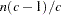, whereis the number of observations andis the number of classes

• Total-sample covariance matrix

• Within-class correlation coefficients and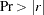to test the hypothesis that the within-class population correlation coefficients are zero

• Pooled within-class correlation coefficients andto test the hypothesis that the partial population correlation coefficients are zero

• Between-class correlation coefficients andto test the hypothesis that the between-class population correlation coefficients are zero

• Total-sample correlation coefficients andto test the hypothesis that the total population correlation coefficients are zero

• Simple statistics, including
(the number of observations), sum, mean, variance, and standard deviation both for the total sample and within each class

• Total-sample standardized class means, obtained by subtracting the grand mean from each class mean and dividing by the total sample standard deviation

• Pooled within-class standardized class means, obtained by subtracting the grand mean from each class mean and dividing by the pooled within-class standard deviation

• Pairwise squared distances between groups

• Univariate test statistics, including total-sample standard deviations, pooled within-class standard deviations, between-class standard deviations, R square,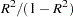,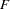, and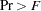(univariatevalues and probability levels for one-way analyses of variance)

By default, PROC CANDISC displays these statistics:

• Multivariate statistics andapproximations including Wilks’ lambda, Pillai’s trace, Hotelling-Lawley trace, and Roy’s greatest root withapproximations, numerator and denominator degrees of freedom (Num DF and Den DF), and probability values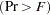. Each of these four multivariate statistics tests the hypothesis that the class means are equal in the population. See the section Multivariate Tests in Chapter 4, Introduction to Regression Procedures, for more information.

• Canonical correlations

• Adjusted canonical correlations (Lawley; 1959). These are asymptotically less biased than the raw correlations and can be negative. The adjusted canonical correlations might not be computable and are displayed as missing values if two canonical correlations are nearly equal or if some are close to zero. A missing value is also displayed if an adjusted canonical correlation is larger than a previous adjusted canonical correlation.

• Approximate standard error of the canonical correlations

• Squared canonical correlations

• Eigenvalues of. Each eigenvalue is equal to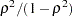, where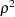is the corresponding squared canonical correlation and can be interpreted as the ratio of between-class variation to pooled within-class variation for the corresponding canonical variable. The table includes Eigenvalues, Differences between successive eigenvalues, the Proportion of the sum of the eigenvalues, and the Cumulative proportion.

• Likelihood ratio for the hypothesis that the current canonical correlation and all smaller ones are zero in the population. The likelihood ratio for the hypothesis that all canonical correlations equal zero is Wilks’ lambda.

• Approxstatistic based on Rao’s approximation to the distribution of the likelihood ratio (Rao; 1973, p. 556; Kshirsagar; 1972, p. 326)

• Numerator degrees of freedom (Num DF), denominator degrees of freedom (Den DF), and, the probability level associated with thestatistic

The following statistics can be suppressed with the SHORT option:

• Total canonical structure, giving total-sample correlations between the canonical variables and the original variables

• Between canonical structure, giving between-class correlations between the canonical variables and the original variables

• Pooled within canonical structure, giving pooled within-class correlations between the canonical variables and the original variables

• Total-sample standardized canonical coefficients, standardized to give canonical variables with zero mean and unit pooled within-class variance when applied to the total-sample standardized variables

• Pooled within-class standardized canonical coefficients, standardized to give canonical variables with zero mean and unit pooled within-class variance when applied to the pooled within-class standardized variables

• Raw canonical coefficients, standardized to give canonical variables with zero mean and unit pooled within-class variance when applied to the centered variables

• Class means on the canonical variablesPrevious Page | Next Page | Top of Page